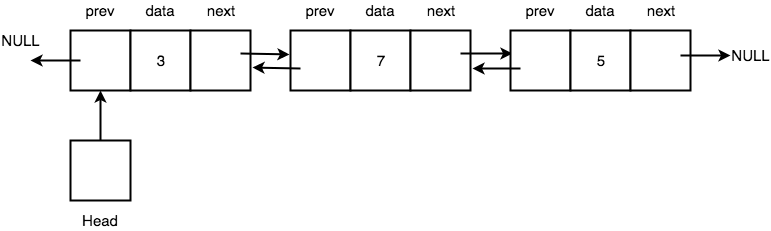# DataStructures Series #8 – The Doubly LinkedList DataStructure

Originally posted on my medium page: https://medium.com/@otarutunde

The Doubly LinkedList Datastructure

The doubly linkedList datastructure is a variation of the linkedList datastructure (Implementation of a List ADT), unlike the singly LinkedList datastructure , the doubly linkedList datastructure flows in both direction. Each node has three cells:

• One contains the address of the previous node
• One contains the data
• One contains the address of the next node.photo credit: studytonight.com

Doubly Linked List Operations:

• Insert (data, position)
• Delete (data)
• Get (data)
• Insert (data)

Insert (data, position) — This will insert a node having the data at the specified position in the list

Delete (data) — This will delete the last node having the data in the list

Get (data) — This fetches the node having the data from the list sequence

Insert (data)— This will add a new node having the data to the end of the list

Implementation

The approach here is to create a class representing a node with its following properties.

Below is a pseudocode implementation for creating a list of 1 <–> 3 <–> 4:

```Class Node {

Node prevNode = null;
int data = null;
Node nextNode = null;

class_constructor(prevNode, data, nextNode) {
this.nextNode = nextNode;
this.data = data;
this.prevNode = prevNode;
}

}

Node four = new Node(null, 4, null);
Node three = new Node(null, 3, four);
Node one = new Node(null, 1, three);
three.prevNode = one;
four.prevNode = three;```

Insert a Node with data value 2 at position 1 (zero indexed) so the new sequence becomes 1 <–> 2 <–> 3 <–> 4:

InsertNode (2, 1)

```Node prevNode = null;
Node currentNode = one;
listCurrentPosition = 0;

while (currentNode is not null) {
if (listCurrentPosition == 1) {
Node dataNode = new Node(prevNode, 2, currentNode);
prevNode.nextNode = dataNode;
currentNode.prevNode = dataNode;
break out of current loop;
}
prevNode = currentNode;
currentNode = currentNode.nextNode;
listCurrentPosition++;
}```

Delete node with data 3 in the list so the sequence becomes 1 <–> 2 <–> 4:

delete (3)

```Node currentNode = one;
prevNode = null;
while (currentNode.nextNode is not null) {
if (currentNode.data == 3) {
Node node = currentNode.nextNode;
prevNode.nextNode = node;
node.prevNode = prevNode;
break out of the while loop;
}
prevNode = currentNode;
currentNode = currentNode.nextNode;
}```

Get node having data 2:

Get (2)

`Node currentNode = one;while (currentNode.nextNode is not null) {   if (currentNode.data == 2) {      return currentNode;   }   currentNode = currentNode.next;}`

Insert node having data 5 into the list so the sequence becomes 1 <–> 2 <–> 4 <–> 5:

Insert (5)

```Node currentNode = four;
Node five = new Node(four, 5, null);
four.nextNode = five;```

Real life Applications

• A two way music playlist — Music playlists with forward and backward buttons.
• A browser web history — Navigating a web browser history

REFERENCES:

## One thought on “DataStructures Series #8 – The Doubly LinkedList DataStructure”

Comments are closed.